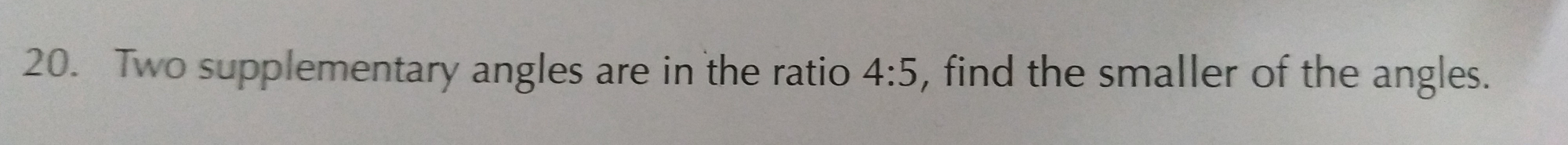"
">

# 20. Two supplementary angles are in the ratio $4: 5,$ find the smaller of the angles."

Given: Two supplementary angles are in the ratio 4:5.

To find: We have to find the smallest of the two angles

Solution:

Two angles are supplementary to each other when their sum is 180 degrees.

Now,

Given that angles are in ratio 4:5.

Let the common factor be = a.

So,

1st angle = 4a

2nd angle = 5a

Also,

4a + 5a = 180o

9a = 180o

a = $\frac{180^{0}}{9}$

a = 20o

Therefore, smaller angle is:

4a = 4 x 20o = 80o

Updated on: 10-Oct-2022

23 Views